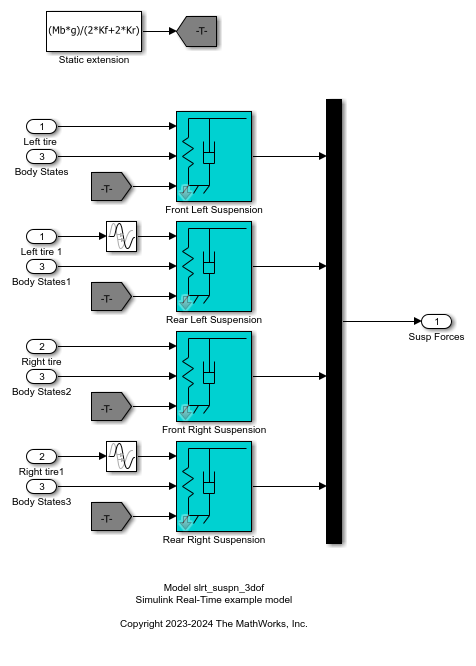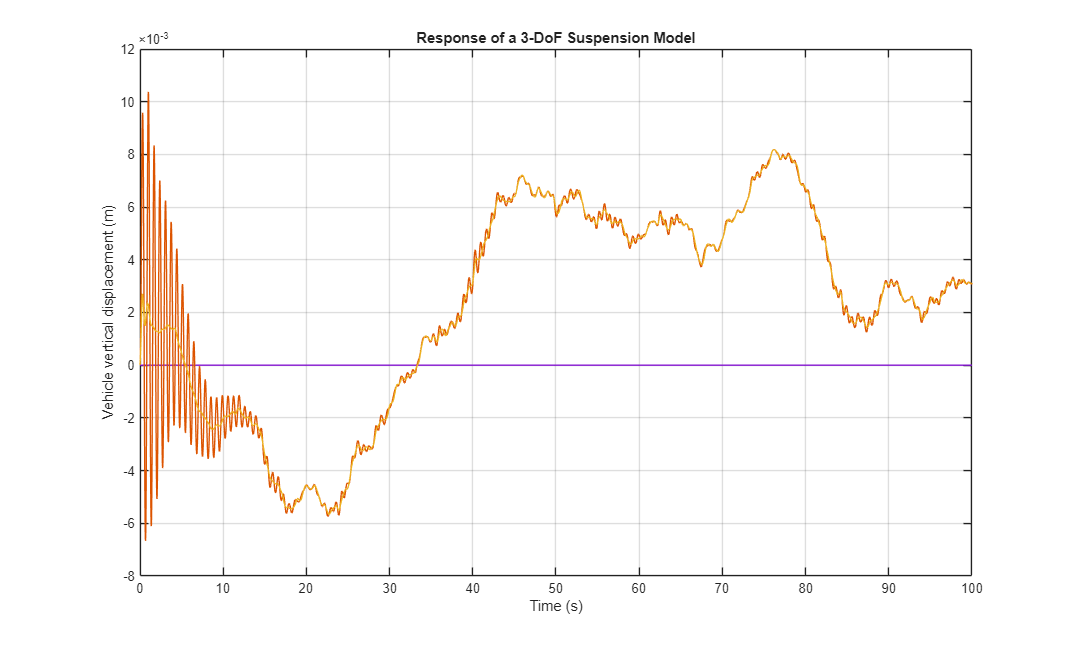# Data Logging with Simulation Data Inspector (SDI)

This example shows how to use a Simulink® Real-Time™ log of signal data and the Simulation Data Inspector. Signals are logged during model execution. At the end of the run, the Simulation Data Inspector interface displays the signal. This example show how to get the signals from the Simulation Data Inspector interface by using the command line.

Open the model `slrt_suspn_3dof`. This model simulates vehicle dynamics based on the interaction between road and suspension for different road profiles.The model captures vehicle dynamics in three degrees of freedom: vertical displacement, roll, and pitch. The Signal Editor block stores measured road profile data for the left and right tires as different test groups. The Road-Suspension Interaction subsystem calculates the suspension forces on the vehicle at the four tire locations based on the road data and the current vehicle state. The Body Dynamics subsystem uses these forces and the resulting pitch and roll moments to calculate the vehicle motion in each of the three degrees of freedom.

Open the model.

```model = 'slrt_suspn_3dof'; mdlOpen = 0; systems = find_system('type', 'block_diagram'); if all(~strcmp(model, systems)) mdlOpen = 1; open_system('slrt_suspn_3dof.slx'); end ```• Configure for a non-Verbose build.

```set_param(model,'RTWVerbose','off'); evalc('slbuild(model)'); ```
• Close the model it is open.

```if (mdlOpen) bdclose(model); end ```

### Run Model to Calculate the Vertical Vehicle Displacement

Using the Simulink Real-Time object variable, `tg`, load and start the model, and modify model parameters.

```tg = slrealtime; load(tg,model); setparam(tg,'','Cf',125); start(tg); while ~strcmp(tg.status,'stopped') pause(5); end stop(tg); ```

### Update Parameters and Re-calculate the Veritical Vehicle Displacement

Using the Simulink Real-Time object variable, `tg`, load and start the model, and modify model parameters

```tg = slrealtime; load(tg,model); setparam(tg,'','Cf',2375); start(tg); while ~strcmp(tg.status,'stopped') pause(5); end stop(tg); ```

### Display Signals in Simulation Data Inspector

To view the plotted signal data, open the Simulation Data Inspector.

```Simulink.sdi.view ```

### Retrieve and Plot Signal Data from Simulation Data Inspector

You can also retrieve the signal data from the SDI and plot the data by using these commands.

• Get all the runs

• Get the run information

• Get the signal.

• Get the signal objects.

• Plot the signals.

The result shows the effect on vehicle dynamics from the interaction between road and suspension for different road profiles.

```runIds = Simulink.sdi.getAllRunIDs(); for i = 1:length(runIds) run = Simulink.sdi.getRun(runIds(i)); signalID = run.getSignalIDsByName('vertical_disp'); if ~isempty(signalID) signalObj = Simulink.sdi.getSignal(signalID); signalArray(:,i) = signalObj.Values(:,1).Data; timeValues = signalObj.Values(:,1).Time; plot(timeValues,signalArray); drawnow; end end grid on; title('Response of a 3-DoF Suspension Model'); xlabel('Time (s)'); ylabel('Vehicle vertical displacement (m)'); ```